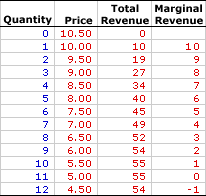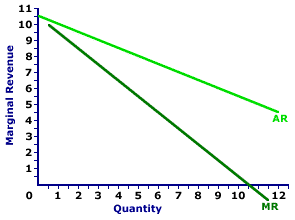Thursday  September 28, 2023
 AmosWEB means Economics with a Touch of Whimsy!AGGREGATE MARKET: An economic model relating the price level and real production that is used to analyze business cycles, gross domestic product, unemployment, inflation, stabilization policies, and related macroeconomic phenomena. The aggregate market, inspired by the standard market model, captures the interaction between aggregate demand (the buyers) and short-run and long-run aggregate supply (the sellers).MARGINAL REVENUE, MONOPOLY:

The change in total revenue resulting from a change in the quantity of output sold. Marginal revenue indicates how much extra revenue a monopoly receives for selling an extra unit of output. It is found by dividing the change in total revenue by the change in the quantity of output. Marginal revenue is the slope of the total revenue curve and is one of two revenue concepts derived from total revenue. The other is average revenue. To maximize profit, a monopoly equates marginal revenue and marginal cost.
Marginal revenue is the extra revenue generated when a monopoly sells one more unit of output. It plays a key role in the profit-maximizing decision of a monopoly relative to marginal cost. A monopoly maximizes profit by equating marginal revenue, the extra revenue generated from production, with marginal cost, the extra cost of production. If these two marginals are not equal, then profit can be increased by producing more or less output.

The relation between marginal revenue and the quantity of output produced depends on market structure. For a perfectly competitive firm, marginal revenue is equal to price and average revenue, all three of which are constant. For a monopoly, monopolistically competitive, or oligopoly firm, marginal revenue is less than average revenue and price, all three of which decrease with larger quantities of output. The constant or decreasing nature of marginal revenue is a prime indication of the market control of a firm.

Marginal revenue can be represented in a table or as a curve. For a perfectly competitive firm, the marginal revenue curve is a horizontal, or perfectly elastic, line. For a monopoly, oligopoly, or monopolistically competitive firm, the marginal revenue curve is negatively sloped.

The marginal revenue received by a monopoly is the change in total revenue divided by the change in quantity, often expressed as this simple equation:

 marginal revenue = change in total revenuechange quantity
Market control means monopoly faces a negatively-sloped demand curve. As such, the price received is not fixed, but depends on the quantity of output sold, and so too does marginal revenue.

### A Table

Marginal Revenue,
MonopolyThe table to the right summarizes the marginal revenue received by a hypothetical firm, Feet-First Pharmaceutical. This firm owns the patent to Amblathan-Plus, the only cure for the deadly, but hypothetical, foot ailment known as amblathanitis. As the only producer of Amblathan-Plus, Feet-First Pharmaceutical is a monopoly with extensive market control, facing a negatively-sloped demand curve. To sell a larger quantity of Amblathan-Plus, Feet-First Pharmaceutical must lower the price.

The first column is the quantity of Amblathan-Plus sold, ranging from 0 to 12 ounces. The second column is the price Feet-First Pharmaceutical receives for selling this medicine, which ranges from \$4.50 to \$10.50 per ounce. The third column is the total revenue Feet-First receives for producing and selling this medicine.

Marginal revenue in the forth column is found by dividing the change in total revenue (from the third column) by the change in quantity (from the first column). For example, when Feet-First Pharmaceutical increases production and sales from 4 ounces of Amblathan-Plus to 5 ounces, its total revenue increases from \$34 to \$40, an increase of \$6. As such, the marginal revenue of producing the fifth ounce of Amblathan-Plus is \$6 (= \$6/1). Each value in the fourth column is calculated in the same way.

One obvious point is that marginal revenue decreases with the quantity of medicine produced. A second point is that marginal revenue is less than the price of Amblathan-Plus for each quantity sold. The price of the fifth ounce of Amblathan-Plus is \$8, but the marginal revenue generated by the fifth ounce is only \$6. Marginal revenue is less than price. And because price is average revenue, marginal revenue is also less than average revenue.

### The Curve

Marginal Revenue Curve,
MonopolyThe marginal revenue curve (MR) for Feet-First Pharmaceutical is displayed in the exhibit to the right. Key to this curve is that Feet-First Pharmaceutical is a monopoly provider of Amblathan-Plus and thus faces a negatively-sloped demand curve. Larger quantities of output are only possible with lower prices.

The vertical axis measures marginal revenue and the horizontal axis measures the quantity of output (ounces of medicine). Although quantity on this particular graph stops at 12 ounces of medicine, it could go higher.

This exhibit displays both the marginal revenue curve (MR) and the average revenue curve (AR), which is also the demand curve. Both are negatively sloped, but the marginal revenue curve lies below the average revenue curve, which means that marginal revenue is less than average revenue (and price) for any given quantity.

Although this marginal revenue curve, and preceding table of marginal revenue numbers, is based on the production activity of Feet-First Pharmaceutical, a well-known monopoly firm, they apply to any firm with market control. Monopolistic competition and oligopoly firms that also face negatively-sloped demand curves generate comparable marginal revenue curves.

### Marginal Revenue and Average Revenue

The preceding table of marginal revenue values and the marginal revenue curve suggest an extremely important point, marginal revenue values are less than the corresponding average revenue values (and price). Selling 5 ounces of Amblathan-Plus, for example, involves a price of \$8. However, the marginal revenue that Feet-First Pharmaceutical receives for increasing the quantity from 4 ounces to 5 ounces is \$6. Other quantities provide comparable results. Marginal revenue is less than price.

How can this be? Why is this so? An explanation seems in order.

A simple explanation is that any decline in an average, which is most certainly happening to average revenue, is associated with a marginal that is less than the average. Because average revenue is decreasing, marginal revenue must be less than average revenue.

A more involved explanation might help.

Consider the situation facing the Feet-First Pharmaceutical monopoly. If Feet-First Pharmaceutical sets the Amblathan-Plus price at \$8.50, then buyers are willing to purchase 4 ounces. However, if Feet-First Pharmaceutical wants to increase the quantity sold from 4 ounces to 5 ounces, then it MUST lower the price from \$8.50 to \$8 per ounce. But here is the catch: Feet-First Pharmaceutical must lower the price for ALL, not just the extra ounce.

What happens to Feet-First Pharmaceutical's total revenue when it lowers the price? Two forces are at work: (1) the revenue gained by adding extra ounces and (2) the revenue lost by lowering the price for existing ounces. Marginal revenue is the net result of both.

• First, by lowering its price, Feet-First Pharmaceutical increases the quantity sold from 4 ounces to 5 ounces. This extra ounce generates an extra \$8 of revenue, the price of the sixth ounce. This is \$8 of extra revenue that Feet-First Pharmaceutical did NOT have at the higher price. If this is all that happens, then Feet-First Pharmaceutical has a marginal revenue of \$8 for the sixth ounce, equal to the price.

• Second, by lowering its price, Feet-First Pharmaceutical collects less revenue from its other 4 ounces. It would have collected \$8.50 per ounce for a total of \$34. But with the lower \$8 price it collects only \$32, a reduction of total revenue by \$2, or \$0.50 per ounce.
The \$8 gained by selling the extra ounce is partially offset by the \$2 lost from lowering the price for other ounces. On net, total revenue increases by only \$6. The loss of revenue collected on existing ounces is the key reason that marginal revenue is less than price.

 <= MARGINAL REVENUE, MONOPOLISTIC COMPETITION MARGINAL REVENUE, PERFECT COMPETITION =>Recommended Citation:

MARGINAL REVENUE, MONOPOLY, AmosWEB Encyclonomic WEB*pedia, http://www.AmosWEB.com, AmosWEB LLC, 2000-2023. [Accessed: September 28, 2023].

Check Out These Related Terms...

Or For A Little Background...

And For Further Study...

Related Websites (Will Open in New Window)...
Search Again?PINK FADFLY[What's This?] Today, you are likely to spend a great deal of time wandering around the downtown area wanting to buy either a replacement nozzle for your shower or a decorative windchime with plastic . Be on the lookout for slightly overweight pizza delivery guys.Your Complete ScopeIt's estimated that the U.S. economy has about \$20 million of counterfeit currency in circulation, less than 0.001 perecent of the total legal currency."The way employees treat customers reflects the manner in which they're treated by management. "-- James Perkins, president, Cornell UniversityWTOWorld Trade OrganizationA PEDestrian's Guide Xtra CreditTell us what you think about AmosWEB. Like what you see? Have suggestions for improvements? Let us know. Click the User Feedback link.| | | | | | | | | | |
| | | |

Thanks for visiting AmosWEB